8 Mar 2014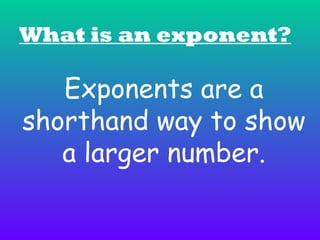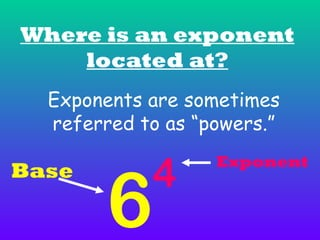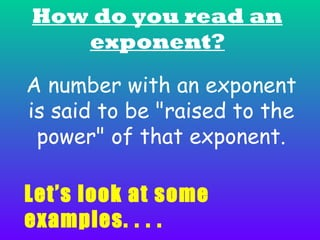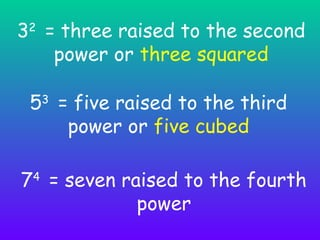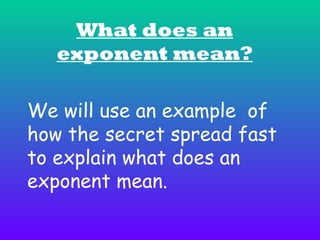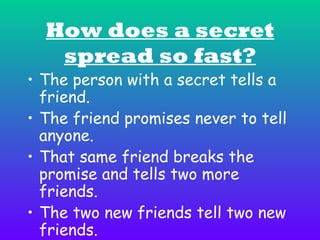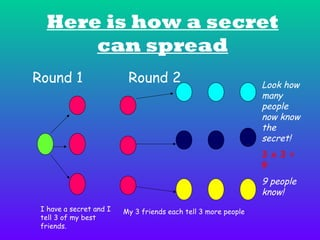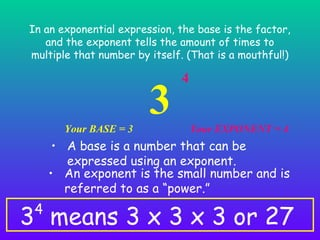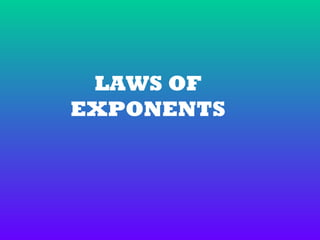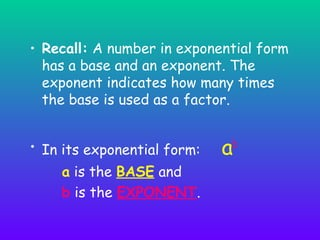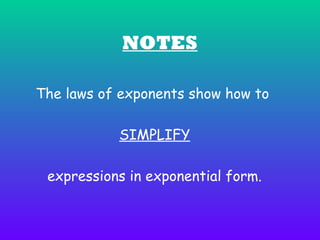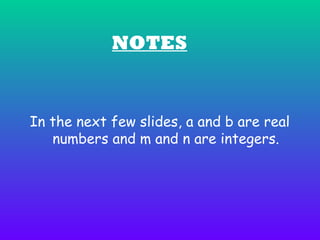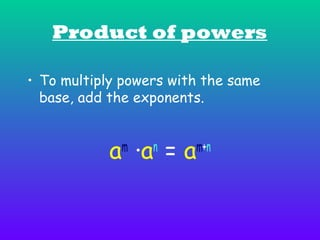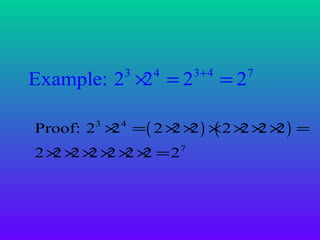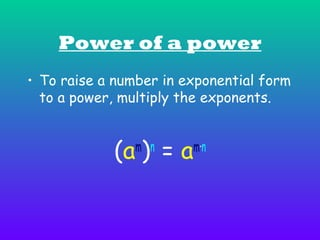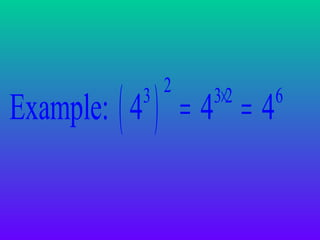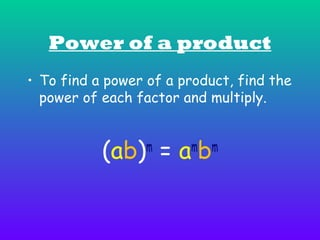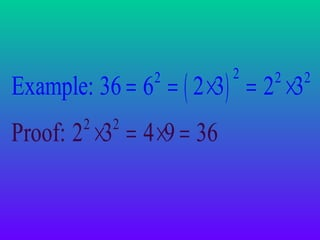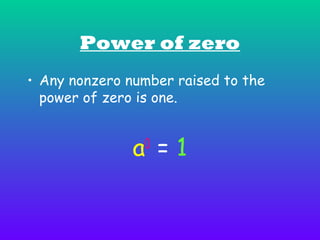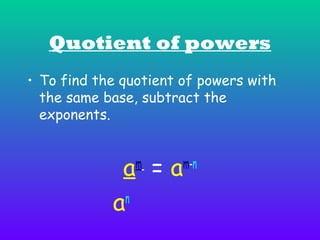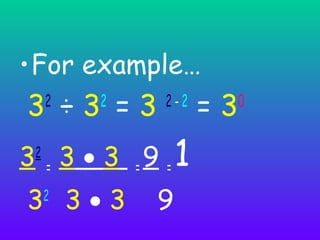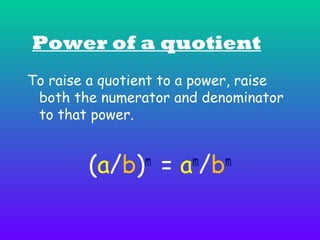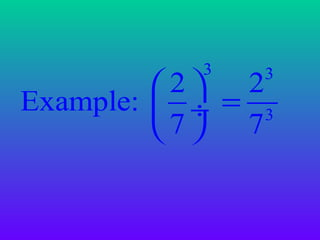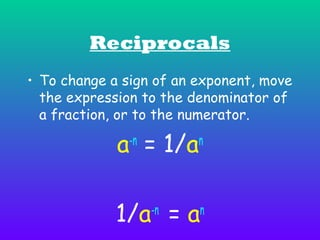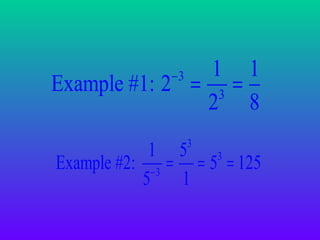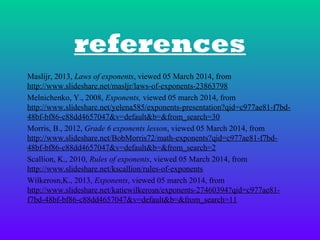1 sur 29

• 2. What is an exponent? Exponents are a shorthand way to show a larger number.
• 3. Where is an exponent located at? Exponents are sometimes referred to as “powers.” Base 6 4 Exponent
• 4. How do you read an exponent? A number with an exponent is said to be "raised to the power" of that exponent. Let’s look at some examples. . . .
• 5. 32 = three raised to the second power or three squared 53 = five raised to the third power or five cubed 74 = seven raised to the fourth power
• 6. What does an exponent mean? We will use an example of how the secret spread fast to explain what does an exponent mean.
• 7. How does a secret spread so fast? • The person with a secret tells a friend. • The friend promises never to tell anyone. • That same friend breaks the promise and tells two more friends. • The two new friends tell two new friends.
• 8. • The two new friends decide to tell two more friends. • This pattern occurs over and over until many people have been told. • By the end of the day it is no longer a secret!
• 9. Here is how a secret can spread Round 1 Round 2 Look how many people now know the secret! 3 x 3 = 9 9 people know! I have a secret and I tell 3 of my best friends. My 3 friends each tell 3 more people.
• 10. In an exponential expression, the base is the factor, and the exponent tells the amount of times to multiple that number by itself. (That is a mouthful!) Your BASE = 3 3 4 Your EXPONENT = 4 • A base is a number that can be expressed using an exponent. • An exponent is the small number and is referred to as a “power.” 3 means 3 x 3 x 3 or 27 4
• 11. LAWS OF EXPONENTS
• 12. • Recall: A number in exponential form has a base and an exponent. The exponent indicates how many times the base is used as a factor. • In its exponential form: a is the BASE and b is the EXPONENT. ab
• 13. NOTES The laws of exponents show how to SIMPLIFY expressions in exponential form.
• 14. NOTES In the next few slides, a and b are real numbers and m and n are integers.
• 15. Product of powers • To multiply powers with the same base, add the exponents. a ∙a = a m n m+n
• 16. Example: 2 × = 2 2 3 4 3+ 4 =2 7 Proof: 23 × 4 = ( 2 × × ) × 2 × × × ) = 2 2 2 ( 2 2 2 2 × × × × × × =2 2 2 2 2 2 2 7
• 17. Power of a power • To raise a number in exponential form to a power, multiply the exponents. (a ) = a m n m∙n
• 18. Example: ( 4 ) = 4 = 4 2 3 3×2 6
• 19. Power of a product • To find a power of a product, find the power of each factor and multiply. (ab) = a b m m m
• 20. Example: 36 = 6 = ( 2 ×3) = 2 ×3 2 Proof: 2 ×3 = 4 ×9 = 36 2 2 2 2 2
• 21. Power of zero • Any nonzero number raised to the power of zero is one. a =1 0
• 22. Quotient of powers • To find the quotient of powers with the same base, subtract the exponents. a =a n a m m-n
• 23. •For example… 3 ÷3 =3 2 2 2-2 3 = 3 • 3 =9 =1 2 3 3•3 2 9 =3 0
• 24. Power of a quotient To raise a quotient to a power, raise both the numerator and denominator to that power. (a/b) = a /b m m m
• 25. 3 3 2 2 Example:  ÷ = 3 7 7
• 26. Reciprocals • To change a sign of an exponent, move the expression to the denominator of a fraction, or to the numerator. a = 1/a -n n 1/a = a -n n
• 27. 1 1 Example #1: 2 = 3 = 2 8 −3 3 1 5 3 Example #2: − 3 = = 5 = 125 5 1
• 28. references Maslijr, 2013, Laws of exponents, viewed 05 March 2014, from http://www.slideshare.net/masljr/laws-of-exponents-23863798 Melnichenko, Y., 2008, Exponents, viewed 05 march 2014, from http://www.slideshare.net/yelena585/exponents-presentation?qid=c977ae81-f7bd48bf-bf86-c88dd4657047&v=default&b=&from_search=30 Morris, B., 2012, Grade 6 exponents lesson, viewed 05 March 2014, from http://www.slideshare.net/BobMorris72/math-exponents?qid=c977ae81-f7bd48bf-bf86-c88dd4657047&v=default&b=&from_search=2 Scallion, K., 2010, Rules of exponents, viewed 05 March 2014, from http://www.slideshare.net/kscallion/rules-of-exponents Wilkerosn,K., 2013, Exponents, viewed 05 march 2014, from http://www.slideshare.net/katiewilkerosn/exponents-27460394?qid=c977ae81f7bd-48bf-bf86-c88dd4657047&v=default&b=&from_search=11
• 29. THE END